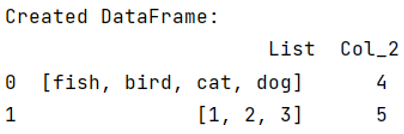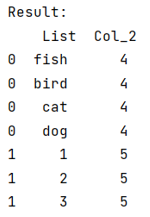# How to create separate rows for each list item where the list is itself an item of a pandas DataFrame column?

Given a Pandas DataFrame where a column is having a list of items, we need to create separate row for each item of columns.
Submitted by Pranit Sharma, on June 01, 2022

We will use pandas.DataFrame.explode() method to achieve this task.

## pandas.DataFrame.explode() Method

This is a special method which is used to convert each item of a column into a separate row, if the elements of a column consists of a list, then for each element of that list, pandas.DataFrame.explode() will create separate row.

Syntax:

```DataFrame.explode(
column,
ignore_index=False
)
```

To work with pandas, we need to import pandas package first, below is the syntax:

```import pandas as pd
```

Let us understand with the help of an example,

```# Importing pandas package
import pandas as pd

# Creating a Dictionary
d = {
'List':[['fish','bird','cat','dog'],[1,2,3]],
'Col_2':[4,5]
}

# Creating a DataFrame
df = pd.DataFrame(d)

# Display DataFrame
print("Created DataFrame:\n",df,"\n")
```

Output:Now, use explode() method.

```# Exploding the lists of column List
result = df.explode('List')

# Display result
print("Result:\n",result)
```

Output:Preparation

What's New

Top Interview Coding Problems/Challenges!